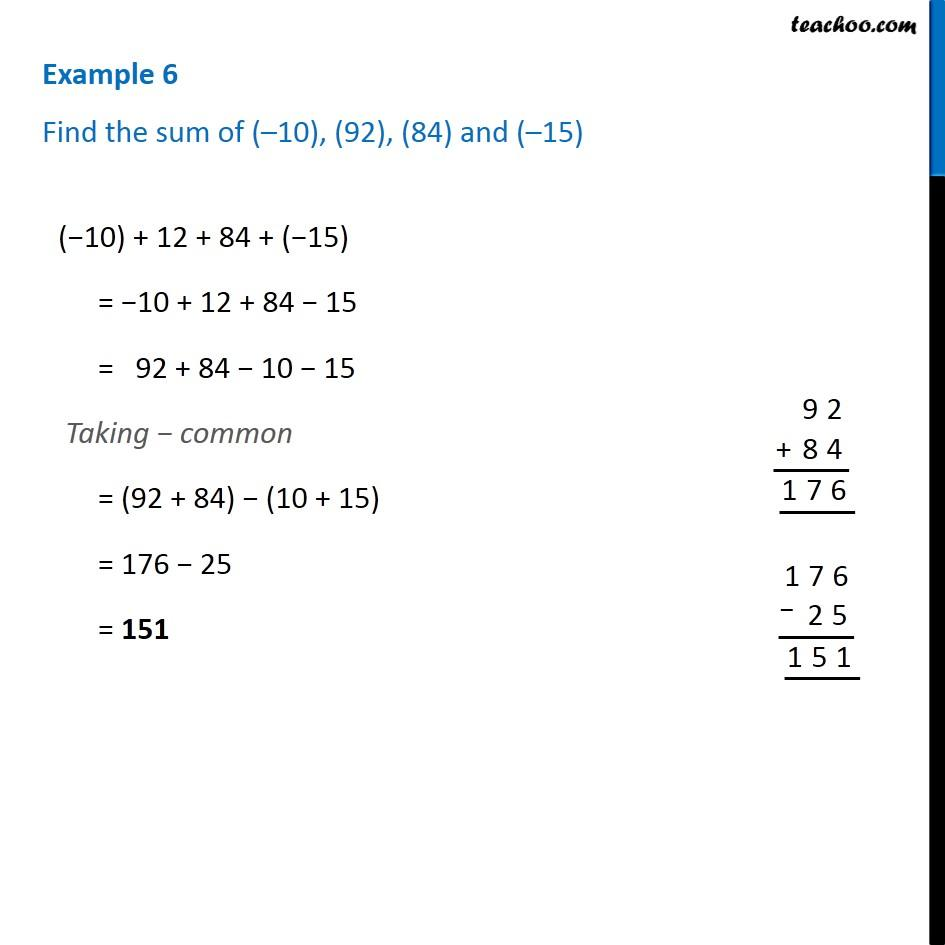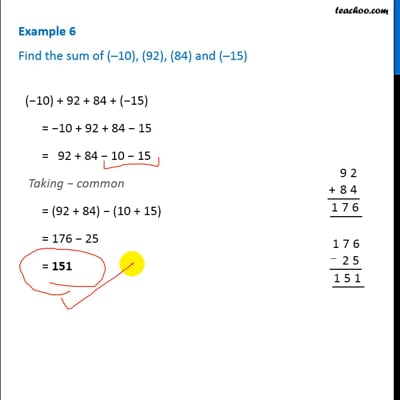Examples

Chapter 6 Class 6 Integers
Serial order wiseThis video is only available for Teachoo black users

Introducing your new favourite teacher - Teachoo Black, at only ₹83 per month

### Transcript

Example 6 - Chapter 6 Class 6 NCERT Book - Integers Find the sum of (–10), (92), (84) and (–15) (−10) + 92 + 84 + (−15) = −10 + 92 + 84 − 15 = 92 + 84 − 10 − 15 Taking − common = (92 + 84) − (10 + 15) = 176 − 25 = 151